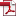• KSII Transactions on Internet and Information Systems
Monthly Online Journal (eISSN: 1976-7277)

# Measure Correlation Analysis of Network Flow Based On Symmetric Uncertainty

Vol. 6, No. 6, June 25, 201210.3837/tiis.2012.06.009, Download Paper (Free):#### Abstract

In order to improve the accuracy and universality of the flow metric correlation analysis, this paper firstly analyzes the characteristics of Internet flow metrics as random variables, points out the disadvantages of Pearson Correlation Coefficient which is used to measure the correlation between two flow metrics by current researches. Then a method based on Symmetrical Uncertainty is proposed to measure the correlation between two flow metrics, and is extended to measure the correlation among multi-variables. Meanwhile, the simulation and polynomial fitting method are used to reveal the threshold value between different correlation degrees for SU method. The statistical analysis results on the common flow metrics using several traces show that Symmetrical Uncertainty can not only represent the correct aspects of Pearson Correlation Coefficient, but also make up for its shortcomings, thus achieve the purpose of measuring flow metric correlation quantitatively and accurately. On the other hand, reveal the actual relationship among fourteen common flow metrics.